### What are your chances of acceptance?Duke UniversityUCLA
Unweighted GPA: 3.7
1.0
4.0
SAT: 720 math
200
800
| 800 verbal
200
800

#### Extracurriculars

Low accuracy (4 of 18 factors)Nisha Desai
10 AP Guides

# 10 Hardest AP Calculus BC Practice Questions

### Do you know how to improve your profile for college applications?

See how your profile ranks among thousands of other students using CollegeVine. Calculate your chances at your dream schools and learn what areas you need to improve right now — it only takes 3 minutes and it's 100% free.

## What’s Covered:

The AP Calculus BC Exam historically has a higher pass rate than other AP exams, with 81.3% of students receiving a score of 3 or higher in 2020 and 44.6% of students receiving a score of 5. To do well on this exam, you’ll need to have an in-depth knowledge of important calculus topics and be prepared to answer many involved questions in a limited time. This blog post will go over some of the more difficult questions that you might encounter on the AP Calculus BC Exam, with detailed explanations of how to solve them.

## How Will AP Scores Impact My College Chances?

AP scores actually have little impact on your college chances. In fact, most college applications don’t require you to report your scores. AP classes (more so than AP exams) boost your application by demonstrating your willingness to challenge yourself academically. You can find out more about the impact of AP scores on your admissions in our blog post.

If you want to see how your AP classes affect your chances at getting into specific colleges, try out CollegeVine’s Admissions Chances Calculator. This free tool will let you know your chances at the schools of your choice by evaluating your test scores, grades, and extracurriculars. It’ll even offer advice to further strengthen your application!## Overview of the AP Calculus BC Exam

The AP Calculus BC Exam will have both paper and digital formats in 2021.

The paper administration is held on May 4, 2021 and May 24, 2021:

• Section I: Multiple Choice, 50% of exam score

• No calculator: 30 questions (60 minutes)

• Calculator: 15 questions (45 minutes)

• Section II: Free Response, 50% of exam score

• Calculator: 2 questions (30 minutes)

• No Calculator: 4 questions (60 minutes)

The digital administration is held on June 9, 2021:

• Section I: Multiple Choice

• 45 questions (1 hour 45 minutes), 50% of exam score

• Section II: Free Response

• 6 questions (1 hour 30 minutes), 50% of exam score

A calculator can be used during all sections of the digital exam.

The AP Calculus BC Exam contains all the concepts on the AP Calculus AB Exam, along with two additional topics:

1. Limits and Continuity (4–7%)
2. Differentiation: Definition and Fundamental Properties (4–7%)
3. Differentiation: Composite, Implicit, and Inverse Functions (4–7%)
4. Contextual Applications of Differentiation (6–9%)
5. Analytical Applications of Differentiation (8–11%)
6. Integration and Accumulation of Change (17–20%)
7. Differential Equations (6–9%)
8. Applications of Integration (6–9%)
9. Parametric Equations, Polar Coordinates, and Vector-Valued Functions (11–12%)
10. Infinite Sequences and Series (17–18%)

The last two topics are specific to the AP Calculus BC Exam.

## 10 Hardest AP Calculus BC Questions

We’ll be focusing on questions specific to the AP Calculus BC Exam (i.e. they wouldn’t be found on the AP Calculus AB Exam). This means that this selection of questions isn’t an accurate representation of the distribution of types of questions that you’ll see on the AP Calculus BC Exam. To see more relevant sample questions, check out our 10 Hardest AP Calculus AB Questions blog post.

### Question 1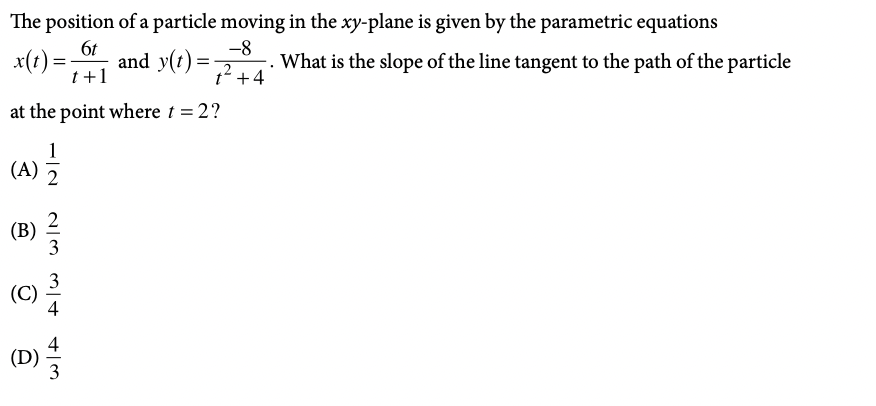This question requires you to compute complicated derivatives. Since it’s asking for the slope of the tangent line, we’ll need to find $$\frac{dy}{dx}$$.

But, we are given equations for $$x$$ and $$y$$ in terms of $$t$$.

This means that if we find $$\frac{dy}{dt}$$ and $$\frac{dx}{dt}$$ at $$t=2$$, we can divide $$\frac{dy}{dt}$$ by $$\frac{dx}{dt}$$ to solve for $$\frac{dy}{dx}$$.

Let’s start by finding $$\frac{dx}{dt}$$ and $$\frac{dy}{dt}$$ by differentiating $$x$$ and $$y$$ with respect to $$t$$:

For $$\frac{dx}{dt}$$, we’ll need to use the division rule:

$$\frac{dx}{dt}=\frac{(t+1)6-6t(1)}{(t+1)^2}=\frac{6t+6-6t}{(t+1)^2}=\frac{6}{(t+1)^2}$$

So, at $$t=2$$, $$\frac{dx}{dt}=\frac{6}{(2+1)^2}=\frac{6}{3^2}=\frac{6}{9}=\frac{2}{3}$$.

Next, we can rewrite $$y(t)$$ as $$y(t)=-8(t^2 +4)^{-1}$$ so that we can use the power rule (and chain rule) to differentiate:

$$\frac{dy}{dt}=-8(-1)(t^2 +4)^{-2}\cdot 2t=\frac{16t}{(t^2 +4)^2}$$.

Then, at $$t=2$$, $$\frac{dy}{dt}=\frac{16(2)}{(2^2 +4)^2}=\frac{32}{8^2}=\frac{32}{64}=\frac{1}{2}$$.

Now we can divide, and we get that:

$$\frac{dy}{dx}=\frac{dy/dt}{dx/dt}=\frac{1/2}{2/3}=\frac{3}{4}$$.

### Question 2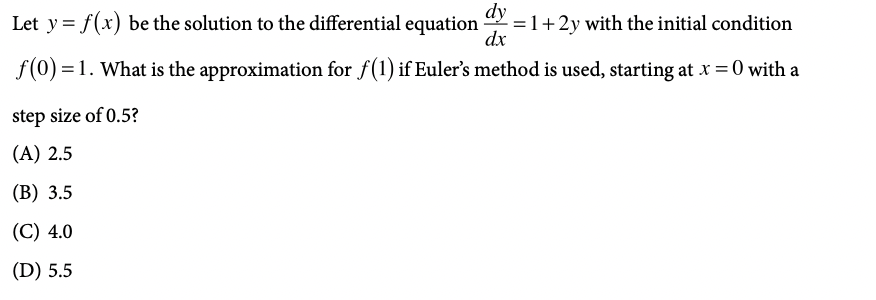Recall that Euler’s method involves the following formula:

$$y_n =y_{n-1}+\Delta x \cdot f'(x_{n-1},y_{n-1})$$

Essentially, what this formula means is that if we’re taking steps of $$\Delta x=1$$, our next $$y$$-value ($$y_n$$) is equal to our previous $$y$$-value ($$y_{n-1}$$) plus the step multiplied by the derivative evaluated at $$y_{n-1}$$ and $$x_{n-1}$$.

So, let’s start with $$y_0 =f(0)=1$$:

$$y_0 =f(0)=1$$

$$y_1=f(0.5)=f(0)+(0.5)f'(0,1)=1+(0.5)(1+2(1))=1+(0.5)(3)=2.5$$

$$y_2=f(1)=f(0.5)+(0.5)f'(0.5,2.5)=2.5+(0.5)(1+2(2.5))=2.5+(0.5)(6)=5.5.$$

Since we’ve reached $$x=1$$, our Euler’s method approximation is $$f(1)=5.5$$.

###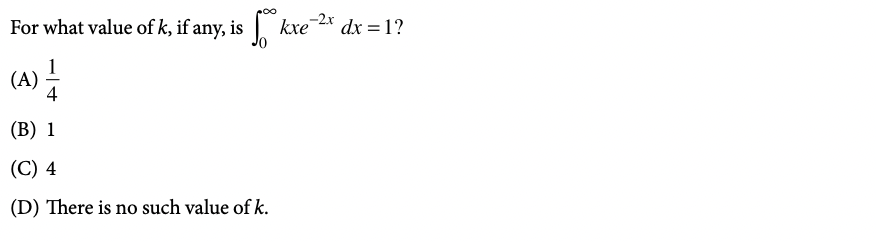To evaluate integrals with infinity, we typically use limits like as follows:

$$\lim_{b \to \infty}\int_{0}^{b} kxe^{-2x}\:dx$$

This way, we can integrate the function first, and then worry about the bounds. For this particular equation, we’ll need to do integration by parts. Also, remember to just treat $$k$$ as a constant.

For integration by parts, remember the formula: $$\int u\:dv= uv-\int v\:du$$

In our case, we can use the following expressions for $$u$$ and $$v$$:

 $$u=kx$$ $$v=\frac{-1}{2}e^{-2x}$$ $$du=k\:dx$$ $$dv=e^{-2x}\:dx$$

Then, $$\int kxe^{-2x} dx=kx(\frac{-1}{2}e^{-2x})-\int \frac{-1}{2}e^{-2x}(k)\:dx$$

$$=\frac{-kxe^{-2x}}{2}-\frac{-k}{2}\int e^{-2x}\:dx$$

$$=\frac{-kxe^{-2x}}{2}+\frac{k}{2}\int e^{-2x}\:dx$$

$$=\frac{-kxe^{-2x}}{2}+\frac{k}{2}(\frac{-1}{2})e^{-2x}$$

$$=-e^{-2x}(\frac{kx}{2}+\frac{k}{4})$$

$$=\frac{-k(2x+1)}{4e^{2x}}$$

So, we can use this expression in our limit:

$$\lim_{b \to \infty}\int_{0}^{b} kxe^{-2x} dx=\lim_{b \to \infty}[\frac{-k(2x+1)}{4e^{2x}}]_{0}^{b}=\lim_{b \to \infty}[\frac{-k(2b+1)}{4e^{2x}}]-[\frac{-k(2(0)+1)}{4e^{2x}}]$$

$$=0-[\frac{-k}{4}]$$

$$=\frac{k}{4}$$.

We wanted this limit to be equal to $$1$$, so we have that $$\frac{k}{4}=1 \Rightarrow k=4$$.

Note that as we were evaluating the limit, the term $$\frac{-k(2b+1)}{4e^{2x}}$$ goes to $$0$$ as $$b$$ goes to infinity since $$e^{2b}$$ grows faster than $$2b$$, so the total expression becomes close to $$0$$.

### Question 4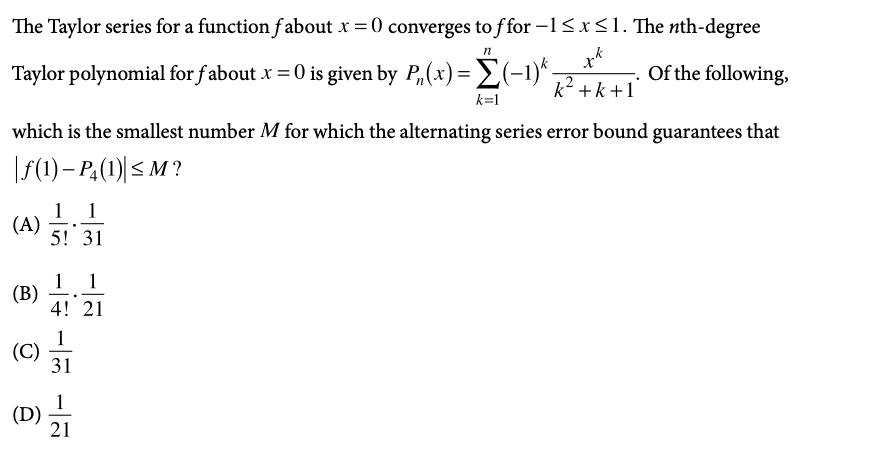This problem becomes fairly simple once you recall that the alternating series error bound guarantees that the error is less than the value of the next term. So, in our case:

$$|f(1)-P_4 (1)|\leq |P_5 (1)|$$

So, we’ll need to calculate $$|P_5 (1)|$$. Since they gave us the Taylor polynomial formula, we can use $$x=1$$ and $$k=5$$.

Then, $$|P_5 (1)|=|(-1)^5 \cdot \frac{(1)^5}{5^2 +5+1}|=|-1\cdot \frac{1}{31}|=1/31$$.

### Question 5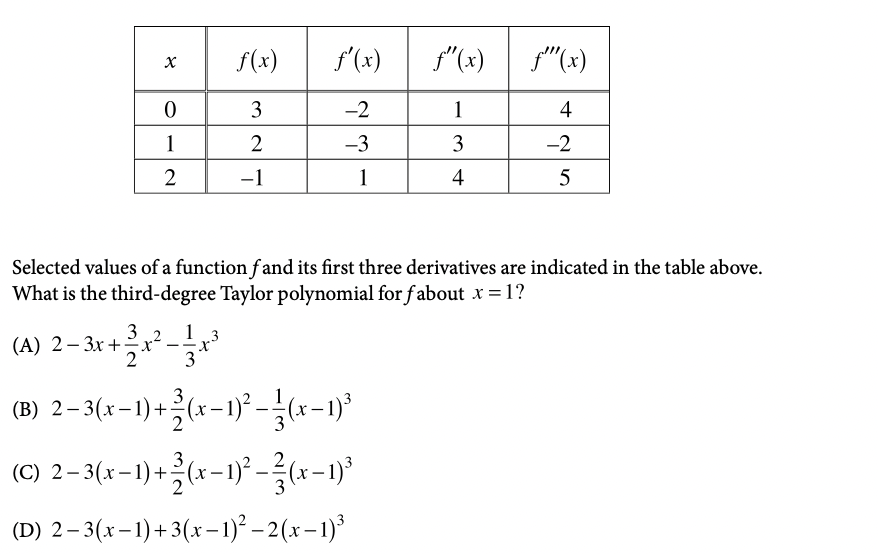You should have the formula for each component of a Taylor polynomial memorized. The $$n$$th component of a Taylor series for $$f$$ about $$x=a$$ is given by:

$$(x-a)^n \frac{f^n (a)}{n!}$$

Since we want the third-degree Taylor polynomial, we’ll need to evaluate for $$n=0, 1, 2,$$ and $$3$$, and we can use the chart to find the values of the derivatives. Be careful to only look at the second row of the chart, since we only care about the values at $$x=1$$:

$$P_3 (x)=(x-1)^0 \frac{f^0 (1)}{0!}+(x-1)^1 \frac{f^1 (1)}{1!}+(x-1)^2 \frac{f^2 (1)}{2!}+(x-1)^3 \frac{f^3 (1)}{3!}$$

$$=1\cdot \frac{2}{1}+(x-1)\frac{-3}{1}+(x-1)^2 \frac{3}{2}+(x-1)^3 \frac{-2}{6}$$

$$=2-3(x-1)+\frac{3}{2}(x-1)^2 -\frac{1}{3}(x-1)^3$$

## Discover your chances at hundreds of schools

Our free chancing engine takes into account your history, background, test scores, and extracurricular activities to show you your real chances of admission—and how to improve them.

[amp-cta id="9459"]

### Question 6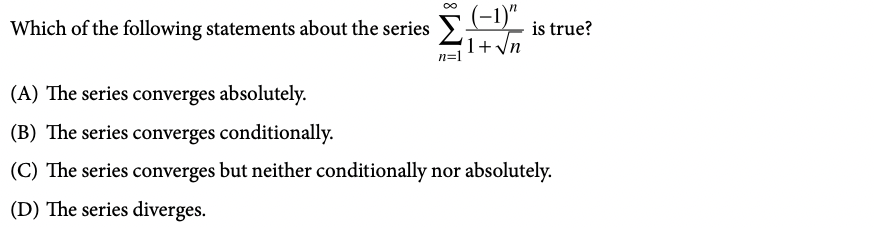For this series to converge absolutely, we’ll need that $$\sum_{n=1}^{\infty}|\frac{(-1)^n}{1+\sqrt{n}}|=\sum_{n=1}^{\infty}\frac{1}{1+\sqrt{n}}$$ converges.

But, the P-series test states that a sum $$\sum_{n=1}^{\infty}\frac{1}{n^p}$$ converges only if $$p>0$$, the absolute value of our series does not converge, since $$p=1/2$$.

So, the series does not converge absolutely and A is incorrect.

Next, we can check if the series converges conditionally. We can do this using the alternating series test. This means that we need to satisfy two conditions:

1. $$\frac{1}{1+\sqrt{n}}$$ is a decreasing sequence

2. $$\lim_{n\to \infty}\frac{1}{1+\sqrt{n}}=0$$

Since both of these conditions are satisfied (as $$n$$ increases, $$\frac{1}{1+\sqrt{n}}$$ decreases and as $$n$$ approaches infinity, $$\frac{1}{1+\sqrt{n}}$$ approaches $$0$$), we have that the alternating series converges conditionally. Therefore, answer choice B is correct.

### Question 7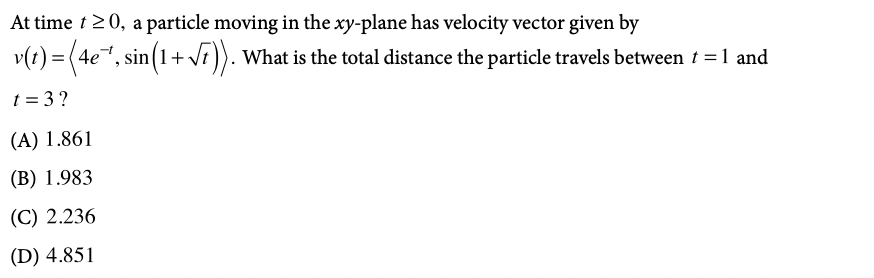The total distance a particle travels in the interval $$[a,b]$$ is given by

$$\int_{a}^{b} \sqrt{(x'(t))^2 +(y'(t))^2}dt$$, where $$x'(t)$$ and $$y'(t)$$ are the derivatives of the components of the position function.

Since most questions involving length of the curve involve some form of differentiation, you might be tempted to take the derivatives of the functions given. But, this question is tricky in that you are actually given the velocity (which is the derivative of position), so there is no need to differentiate further. This means that you can directly plug the given components into the formula:

We use our calculator to determine that $$\int_{1}^{3} \sqrt{(4e^{-t})^2 +(\sin{1+\sqrt{t}})^2}\approx 1.861$$.

### Question 8This question is more conceptual than calculation-based. The first thing to note is that if the integral converges, we must have that the series converges as well. Therefore, answer choice D is incorrect.

Next, since the graph of g is given, and we know that it’s positive and decreasing, we know that the sum of the series must be greater than the value of the integral. The following picture helps illustrate this.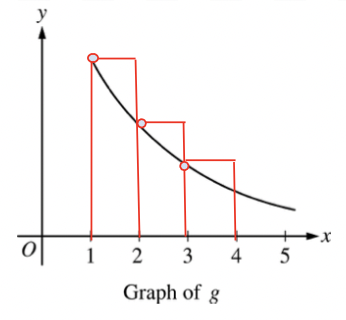Since we have $$a_n =g(n)$$ for each term of the series, the sum of the areas of the red bars is equal to the sum of the series. This is because each height of the bar corresponds to $$g(n)$$, and the widths of each bar is $$1$$, so the sum of the areas of the bars is $$g(1)+g(2)+g(3)+…=a_1 +a_2 +a_3 …$$, which is equivalent to the sum of the series.

But, as we can see from the picture, the area of the bars overestimates the area under the curve, so the sum of the series is greater than the integral.

So, $$\sum_{n=1}^{\infty} a_n > 8$$. This means that answer choice C is the only possible option.

###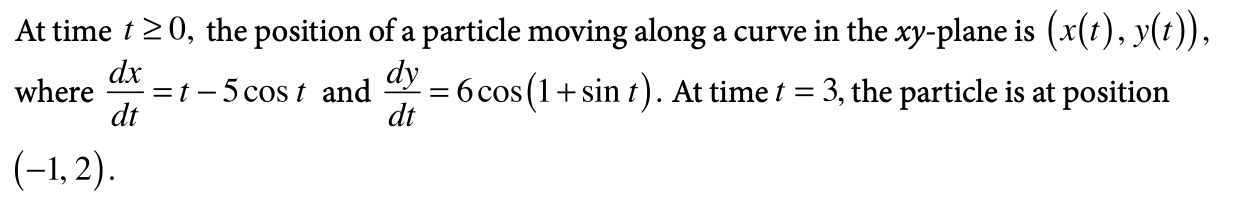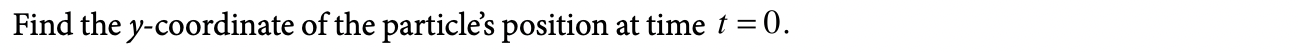This question focuses on the y-coordinate, so we’ll only need to look at $$\frac{dy}{dt}$$.

We know that $$\int_{0}^{3} dy= y(3)-y(0)$$.

Since we want $$y(0)$$, we can rewrite our equation as $$y(0)=y(3)-\int_{0}^{3}\:dy$$.

We are given that $$\frac{dy}{dt}=6\cos{1+\sin{t}}$$ and $$y(3)=2$$. We can find that $$dy=6\cos{1+\sin{t}} dt$$.

So, using our calculator, we get that $$y(0)=y(3)-\int_{0}^{3} 6\cos{1+\sin{t}} dt=2-(-1.634)=3.634.$$

### Question 10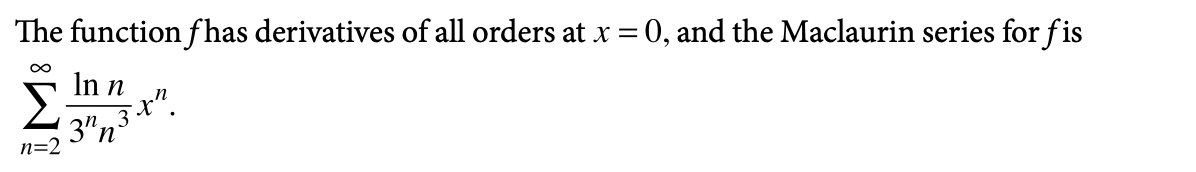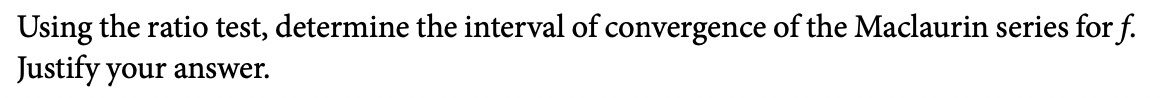Answer: -3 $$\leq$$ x $$\leq$$ 3

The ratio test involves taking the limit of $$|\frac{a_{n+1}}{a_n}|$$ as $$n$$ approaches infinity. If the limit is less than $$1$$, the series converges. If the limit is greater than $$1$$, the series diverges. Finally, if the limit equals $$1$$, the test is inconclusive.

Let’s conduct the ratio test for this problem (remember to treat $$x$$ as a constant):

$$\lim_{n \to \infty} |\frac{a_{n+1}}{a_n}|=\lim_{n \to \infty}{|\frac{\ln{n+1}\cdot x^{n+1}}{3^{n+1}(n+1)^3}|\div |\frac{\ln{n}\cdot x^n}{3^n n^3}|}$$

$$=\lim_{n \to \infty}|\frac{\ln{n+1}\cdot x^{n+1}}{3^{n+1}(n+1)^3}|\times |\frac{3^n n^3}{\ln{n}x^n}|=\lim_{n \to \infty}|\frac{\ln{n+1}}{\ln{n}}\cdot \frac{3^{n}}{3^{n+1}}\cdot (\frac{n}{n+1})^3 \cdot \frac{x^{n+1}}{x^n}|$$

Remember that we can take the limits of products separately:

$$\lim_{n \to \infty} |\frac{\ln{n+1}}{\ln{n}}|\cdot \lim_{n \to \infty} |\frac{3^n}{3^{n+1}}|\cdot \lim_{n \to \infty} |(\frac{n}{n+1})^3|\cdot \lim_{n \to \infty} |\frac{x^{n+1}}{x^n}|$$

$$=\lim_{n \to \infty}1 \cdot \lim_{n \to \infty}1/3 \cdot \lim_{n \to \infty}1 \cdot \lim_{n \to \infty} |x|$$

If we simplify, we get $$\lim_{n \to \infty} |\frac{a_{n+1}}{a_n}|=|\frac{x}{3}|$$, and since we want the series to converge, this limit needs to be less than $$1$$.

So, $$|\frac{x}{3}|<1$$. This becomes $$-1\lt \frac{x}{3}\lt 1$$, or $$-3\lt x\lt 3$$.

But, we’re not done here! We need to check the end points to see if the series converges when $$x=-3$$ or $$x=3$$.

At $$x=-3$$, we have $$\sum_{n=2}^{\infty}\frac{\ln{n}}{3^n n^3}(-3)^n=\sum_{n=2}^{\infty}(-1)^n \cdot \frac{\ln{n}}{n^3}$$.

To see if the series converges, we use the alternating series test:

1. $$\frac{\ln{n}}{n^3}$$ decreases as $$n$$ gets larger (since $$n^3$$ is always larger than $$\ln{n}$$)

2. To calculate $$\lim_{n \to \infty}\frac{\ln{n}}{n^3}$$, we can use L’Hospital’s (since both the numerator and denominator approach $$\infty$$):

$$\lim_{n \to \infty}\frac{\ln{n}}{n^3}=\lim_{n \to \infty}\frac{1/n}{3n^2}=\lim_{n \to \infty}\frac{1}{3n^3}=0$$

Since both conditions are satisfied, the series converges at $$x=-3$$.

Next, we can check for convergence at $$x=3$$.

Our series becomes $$\sum_{n=2}^{\infty}\frac{\ln{n}}{3^n n^3}3^n=\sum_{n=2}^{\infty}\frac{\ln{n}}{n^3}$$.

We can use the direct comparison test to determine if the series converges. If we find a bigger series that converges, the direct comparison test states that our series also converges.

So, since we know that $$\ln{n}\lt n$$, we have that:

$$\frac{\ln{n}}{n^3}\lt \frac{n}{n^3}=\frac{1}{n^2}$$

Also, we know that $$\sum_{n=2}^{\infty}\frac{1}{n^2}$$ converges by the P-series test ($$p=2$$, which is greater than $$1$$).

Therefore, $$\sum_{n=2}^{\infty}\frac{\ln{n}}{n^3}$$ also converges, and both $$-3$$ and $$3$$ are included in the interval.

So, our interval of convergence is $$-3\leq x\leq 3$$.

## Final Tips

As you study for this exam, keep these helpful tips in mind:

### Focus on topics specific to AP Calculus BC

Though you’ll obviously need strong knowledge of AP Calculus AB content to do well on this exam, keep in mind that the multiple-choice section of the exam places a greater weight on BC-specific topics. So, make sure you’re confident with parametrizations, polar coordinates, sequences, and series.

However, even if you’ve already taken the AP Calculus AB exam, don’t completely neglect AB topics. Many AP Calculus BC topics build off of AB topics (for example, limits play a big role in series convergence tests), so though you should focus your studying on the former, make sure to brush up on the latter as well.

While it’s obvious that doing calculations in your calculator rather than your head will save you time and help eliminate careless mistakes, there are many other helpful features on a graphing calculator. For example, if you’re asked to compute the volume created by rotating an area around an axis, graph both the function and the bounds. This way you can find the intersections with your calculator rather than solving by hand.

Essentially, there are various calculator tricks that can simplify a tedious problem. It’ll take practice, but the more you use your calculator, the more comfortable you’ll eventually be with using it, which will make the test-taking process go much more quickly and smoothly.

Take a look at some of CollegeVine’s other guides to AP exams:Short Bio
Nisha Desai is a second year student at the University of California, Riverside. She recently started working at CollegeVine, but has done application guidance and tutoring in a private capacity for a couple of years. She is in school to eventually get her Masters in Education and enjoys reading and running in her free time.